## Free Mechanical Reasoning Test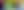# Mechanical Reasoning Tests

Updated 16 November 2021

## What Are Mechanical Reasoning Tests?

Mechanical reasoning tests (also known as mechanical comprehension or mechanical ability tests) measure your knowledge of straightforward mechanical and physical concepts.

They do not measure your underlying mechanical aptitude in the same way that abstract reasoning questions measure your underlying intellectual ability.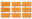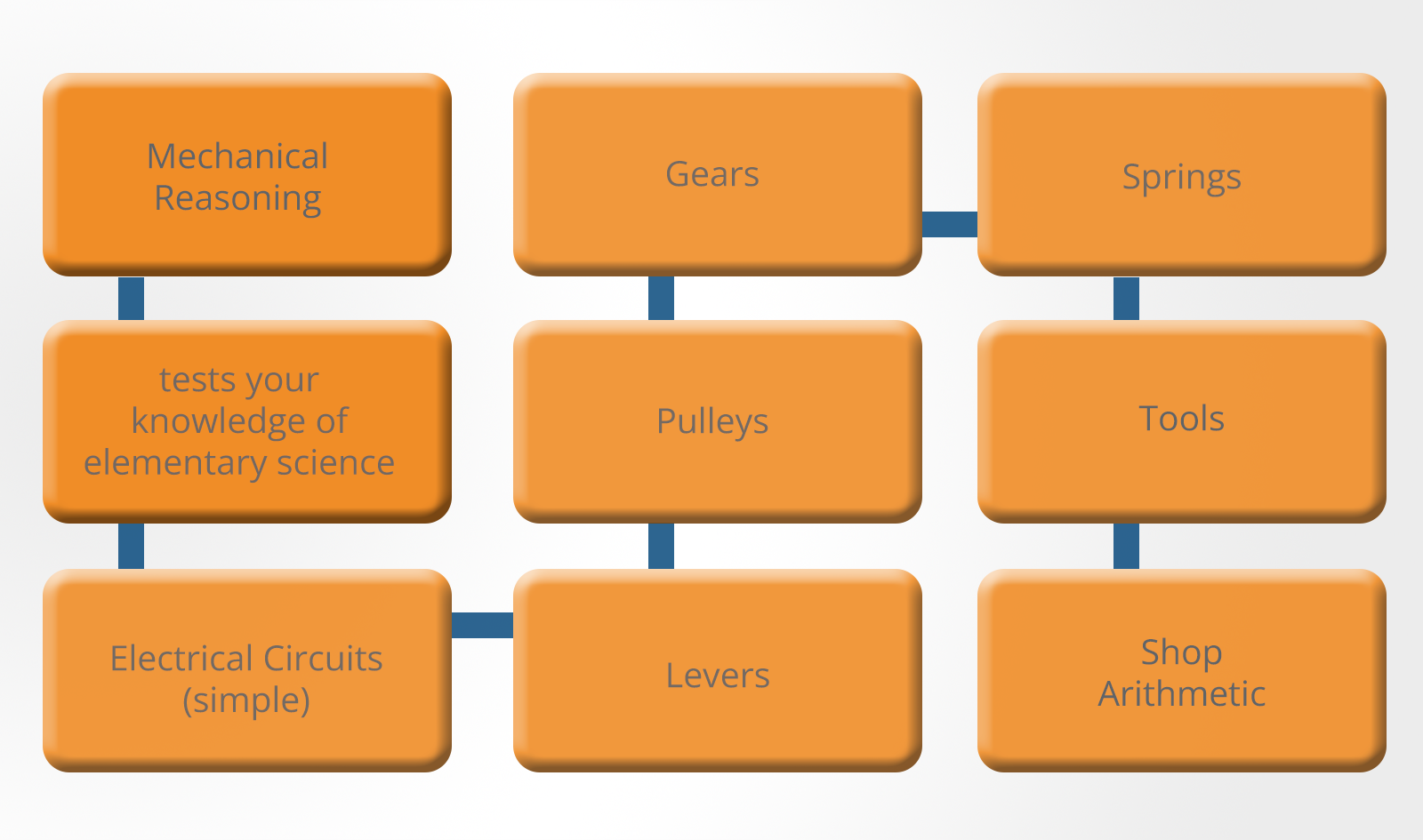Mechanical Reasoning Tests

You could sit an abstract reasoning test without having seen one before and still get a reasonable score.

The same is not usually true of mechanical reasoning where your score will depend significantly on your knowledge of:

You may have come across many of these topics in elementary science and the questions on these topics are fairly straightforward.

If elementary science classes seem like a long time ago then you may need to refresh your memory.

## Different Suppliers of Mechanical Reasoning Tests

### Bennett Test of Mechanical Comprehension

The Bennett Test of Mechanical Comprehension has been used for more than 50 years by employers and schools to evaluate an individual’s ability to solve basic mechanical problems.

Most commonly, this test is taken as part the assessment process for businesses or organizations that are hiring into mechanically-related positions.

Specifically, the test serves to evaluate how well an individual can identify the relationship between physical forces and various mechanical elements in the world around them.

The test focuses on making sure that an individual:

• Understands the function of basic tools
• Has good spatial perception
• Can quickly learn and apply basic mechanical principles

You will be expected to complete a total of 68 questions in 30 minutes. This means you will have less than 30 seconds per question.

The test is written at around a sixth-grade level so you don’t need to have higher education qualifications to be able to complete it. The Bennett test tries to find the level of natural mechanical thinking that the test taker possesses.

The test has been proven to do an excellent job of predicting work performance, therefore it has remained popular for use in a wide variety of fields.

### Barron’s Test of Mechanical Aptitude

At one time, Barron’s Test of Mechanical Aptitude was the most commonly used mechanical reasoning test in the world.

It has its origins in the armed services and was seen as the gold standard for measuring the mechanical reasoning abilities of those looking for a job or a military position.

It tests concepts such as:

• Gears
• Pulleys
• Line-following
• Matching shapes
• Hole punching, and more

Modern versions of this test are usually taken on a computer but a pencil and paper option is also available.

While the test is usually used as a screening process for entry into various military careers, it is also useful for individuals wanting to assess their strengths and weaknesses when making career decisions.

### Stenquist Test of Mechanical Aptitude

The Stenquist Test of Mechanical Aptitude is one of the oldest mechanical reasoning tests still in use today.

Dating back more than 100 years, the principles of this test have not changed much in that time.

The Stenquist test measures mechanical aptitude differently from standard mechanical tests.

The test requires candidates determine which picture best fits with another group of pictures.

Those with a strong inherent knowledge of machines and tools will succeed on this test without much (if any) formal training or education.

Since the test relies on pictures to evaluate the individual, any reading or comprehension issues that could create a problem on other tests will not be a factor when taking the Stenquist test.

### Wiesen Test of Mechanical Aptitude

The Wiesen Test of Mechanical Aptitude seeks to determine the test taker’s level of mechanical reasoning and logic.

While the Wiesen test is considered one of the newer tests available for measuring mechanical aptitude, it has already been widely accepted and is used by many different employers.

There are several applications for this test, from personal career planning to screening potential candidates for a variety of positions.

The test is multiple-choice and you will have 30 minutes to answer 60 questions. Not all candidates will be able to complete the test in the time allowed.

The Wiesen test will assess your innate mechanical thinking rather than your educational background or experience.

You will be tested on topics such as:

• Basic machines
• Gravity
• Electricity
• Heat transfer
• Physical properties
• Movement, and more

## Which Employers Use Mechanical Reasoning Tests?

A variety of different organizations use mechanical reasoning tests to assess candidates as part of their screening process.

The test will assess whether a candidate has good spatial and mechanical skills.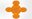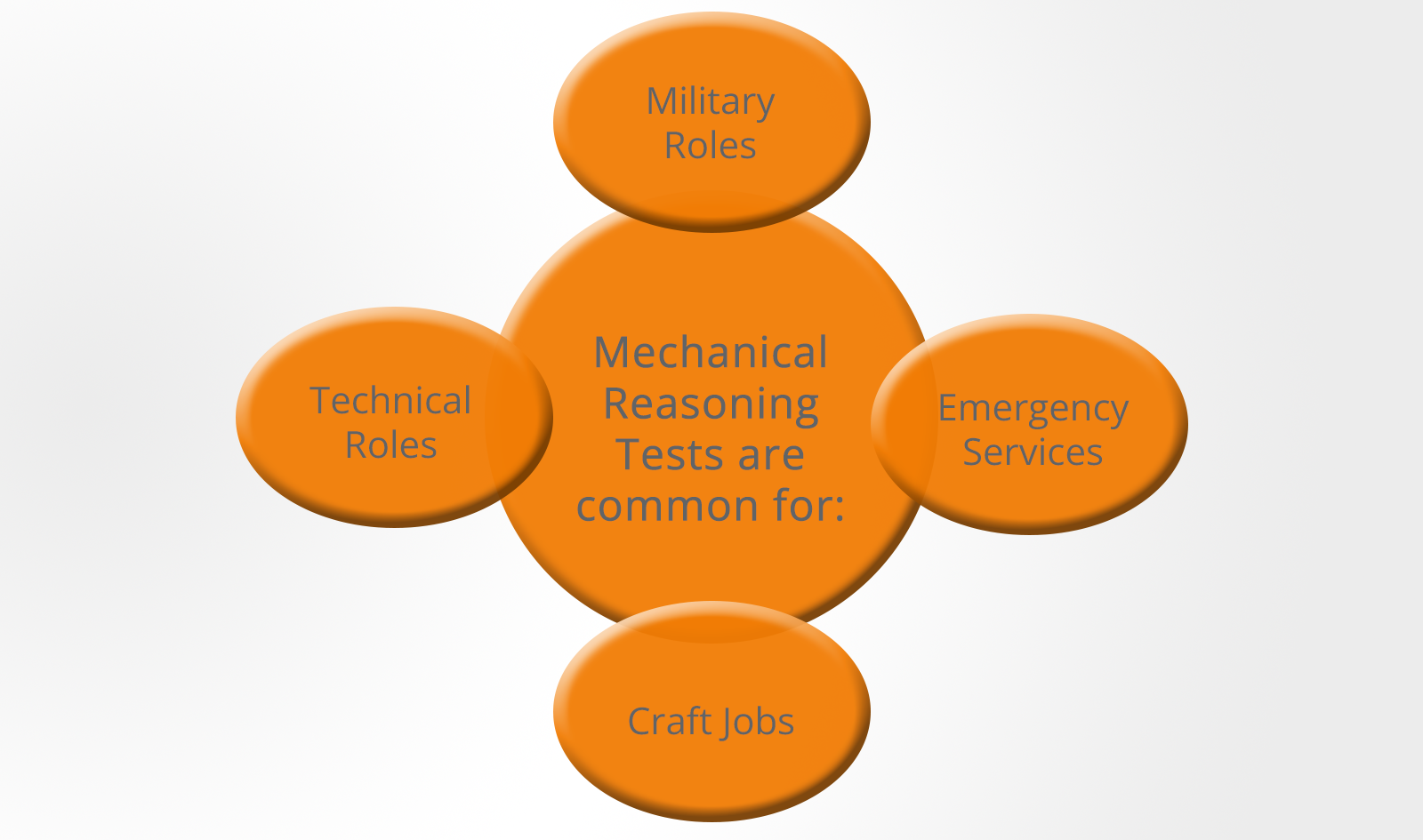Mechanical Reasoning Tests

Some examples of jobs that have been known to use these tests include:

• Firefighter
• Police officer
• Tradesman (such as electrician or plumber)
• Engineer
• Military personnel
• Manufacturing staff
• Production staff
• Many other craft and technical roles

Modern mechanical reasoning tests often include fault diagnosis questions which are used for technical roles that involve finding and repairing faults in electronic control systems.

This type of test is used extensively to select technical and maintenance personnel as well as to select for artificer (technical) roles within the armed forces where the ability to approach problems logically to find the cause of the fault is increasingly important.

## What to Expect on a Mechanical Reasoning Test

When taking a mechanical reasoning test, you will be presented with different mechanical scenarios and you will need to choose the correct answer from the options given. These tests are usually multiple-choice with a fairly short time limit.

While the specific contents and difficulty of the test will vary based on the position that is being applied for, the concepts of gears, levels, tools and more are very likely to be present.

Many of the questions in mechanical reasoning tests will be industry-specific but be prepared for a wide range of topics to be included.

If you are taking a mechanical reasoning test as part of the selection process for the emergency services or the military then the questions you can expect will tend to concentrate on principles rather than on making calculations.

For example, you may be shown three diagrams of a lever and asked which one is the most efficient.

If, however, you are taking a test for a craft or technical job, you may be expected to calculate the actual force required to move a particular lever.

In this case, knowing the principle is not enough, you need to know the formula.

You may also be asked some questions about tools and how they are used as well as some shop arithmetic questions.

These questions approximate the type of reasoning and maths that are needed to estimate the cost of materials, etc.

## Mechanical Reasoning Question Types

### Shop Arithmetic

Some of the questions you will come across may require knowledge of basic mathematics, such as fractions, decimals, ratios, percentages and averages.

These basic mathematical skills are an important aspect of mechanical ability.

You will be expected to make basic calculations based on the information provided and your knowledge of mechanical principles.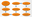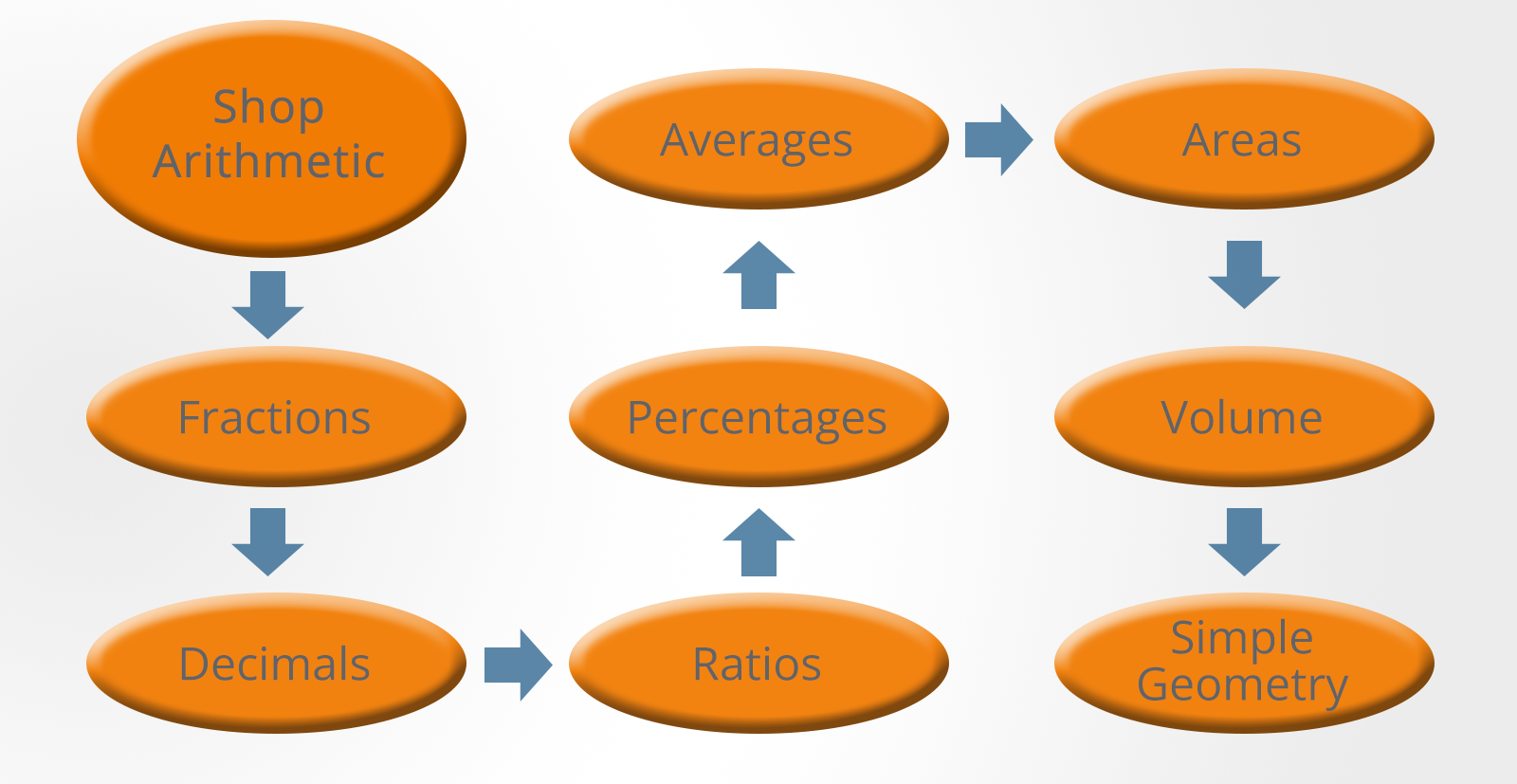Mechanical Reasoning Tests

You will need to know the formulae for calculating the areas and volumes of simple shapes as well as demonstrating familiarity with imperial units including inches, feet and yards.

You should also make sure you understand types of energy and forces and their related equations, such as:

• Potential energy
• Kinetic energy
• Acceleration
• Gravity
• Friction
• Pressure

Some useful formulae include:

Areas of shapes: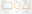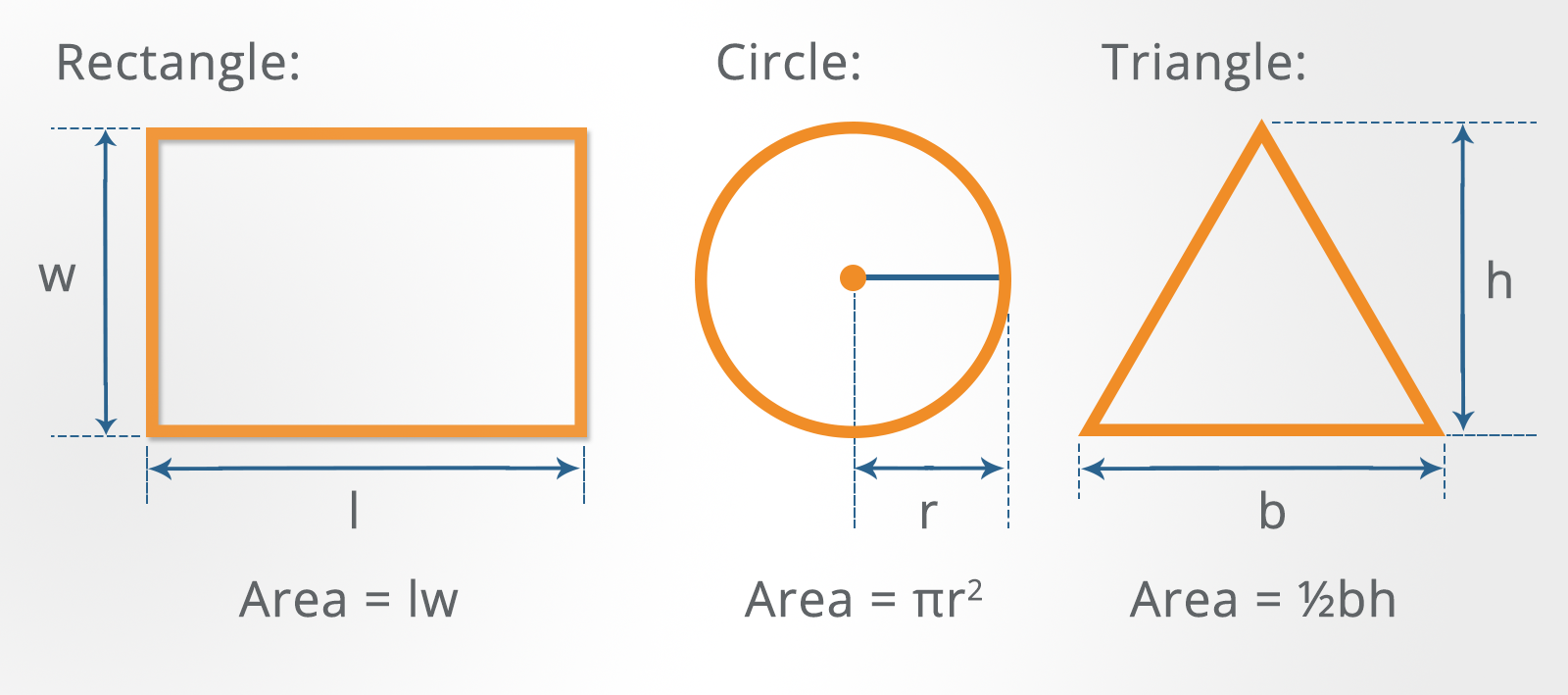Mechanical Reasoning Tests

The volume of rectangular solids and cylinders: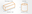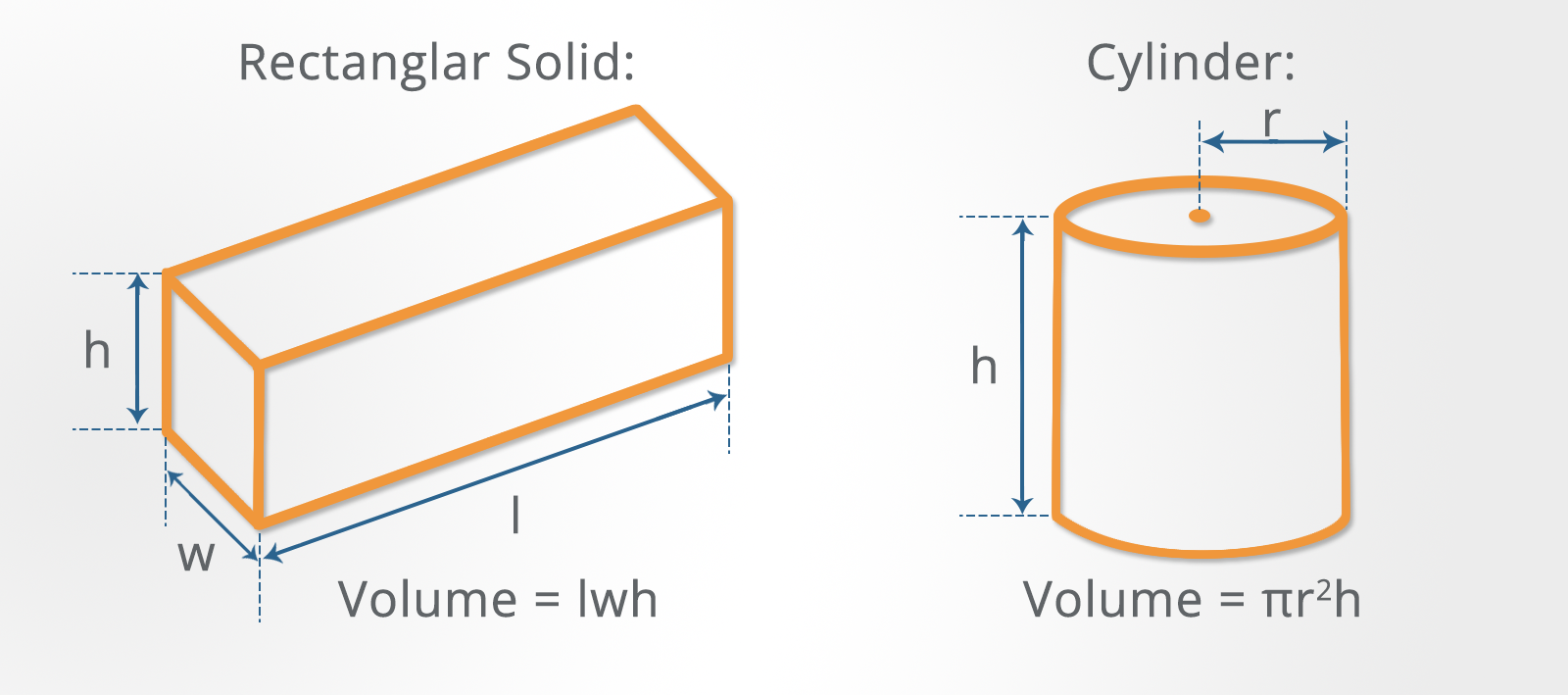Mechanical Reasoning Tests

Simple geometry like Pythagoras Theorem: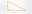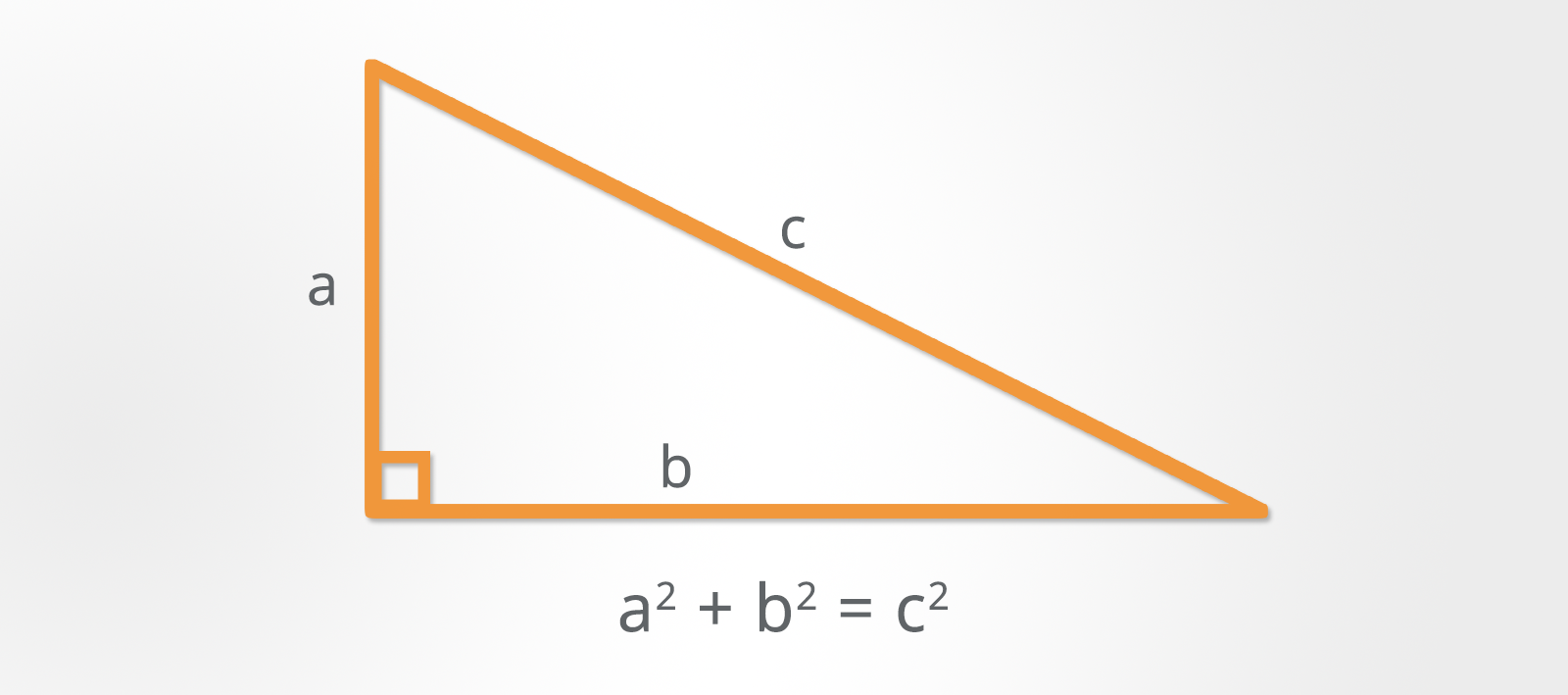Mechanical Reasoning Tests

Example questions: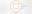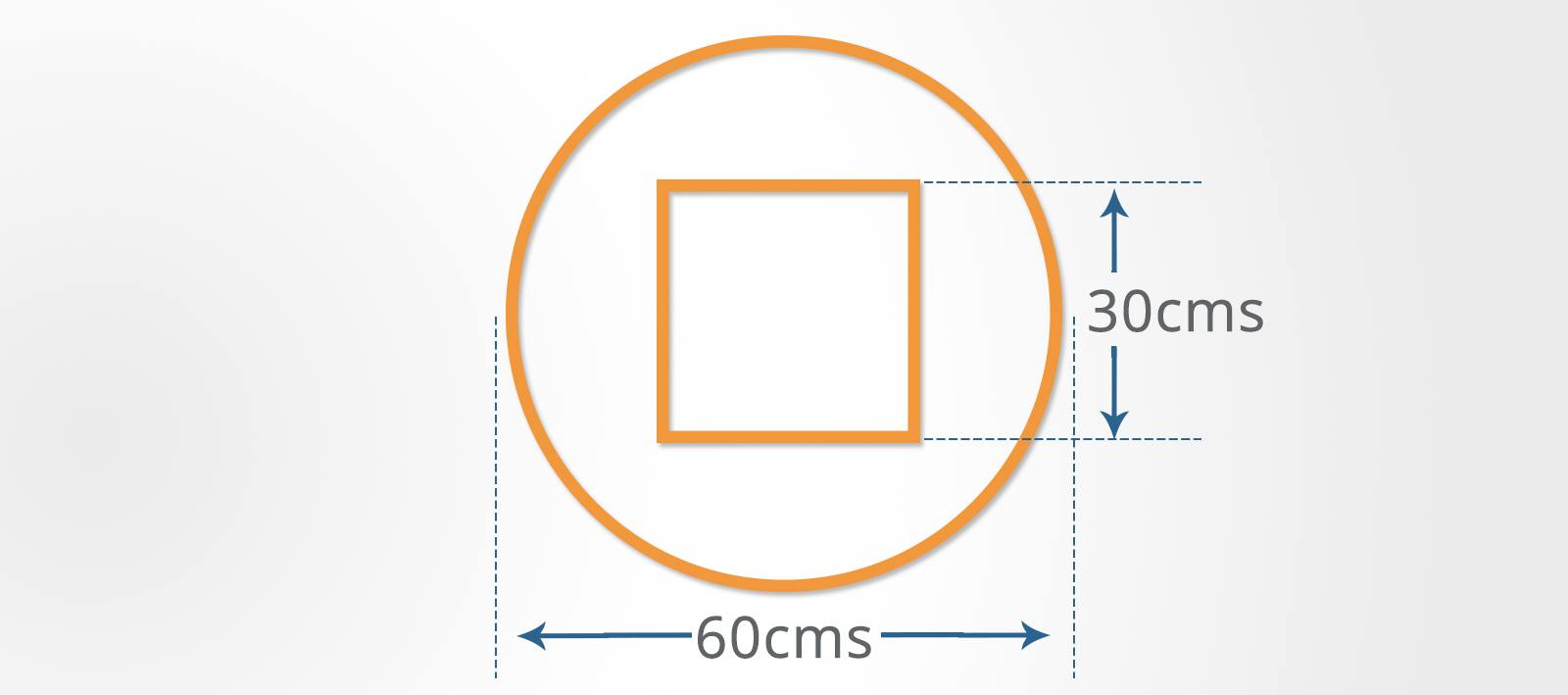Mechanical Reasoning Tests

The sketch above shows a component which is stamped out of sheet steel.

The square in the center is discarded.

These components are stamped out of a continuous steel coil with a width of 70 cms.

The stamping process requires a gap of 25 mm between each component.

The steel coil is supplied in lengths of 25 meters costing \$200.

1. What is the approximate area of the remaining shape in square centimeters?

A) 1,938
B) 1,855
C) 1,926
D) 1,998
E) 1,760

2. What is the approximate percentage of steel wasted including the center square?

A) 56%
B) 50%
C) 62%
D) 48%
E) 52%

3. Assuming minimal wastage, how many components can be produced from each 25-meter coil?

A) 38
B) 40
C) 36
D) 42
E) 37

4. What is the approximate cost of a component if the scrap is sold at 50% of cost?

A) \$3.60
B) \$3.15
C) \$3.55
D) \$5.00
E) \$4.85

1. C
2. A
3. B
4. C

### Levers

A lever consists of a bar that pivots at a fixed point known as the fulcrum. In practice, levers are used to reduce the force needed to move an object; in other words, to make the task easier.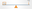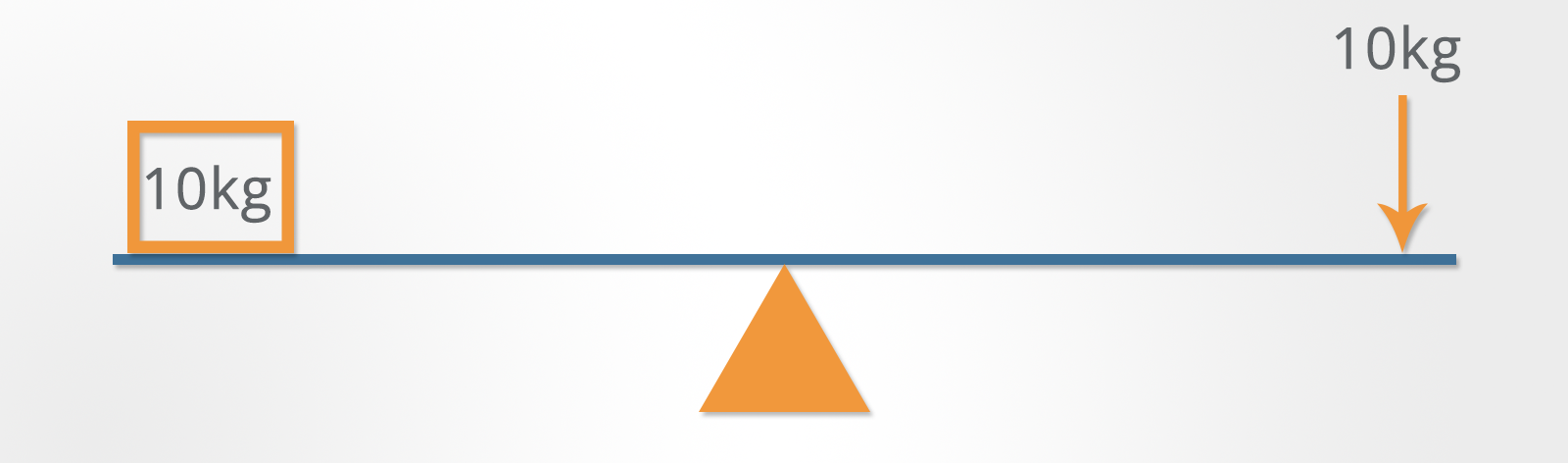Mechanical Reasoning Tests

In the example shown, the fulcrum is at the center of the lever.

This lever provides no mechanical advantage as the force needed to lift the weight is equal to the weight itself.

However, in mechanical reasoning questions, you may see questions where the fulcrum has been placed closer to the force than the weight.

This will mean that a force greater than the weight will be required to lift it.

If you want to lift a weight that is heavier than the force applied, you can move the fulcrum closer to the weight to be lifted.

This affects the force required in the following way:

w x d1 = f x d2

where:

w = weight
d1 = distance from fulcrum to weight
f = force needed
d2 = distance from the fulcrum to the point where force is applied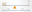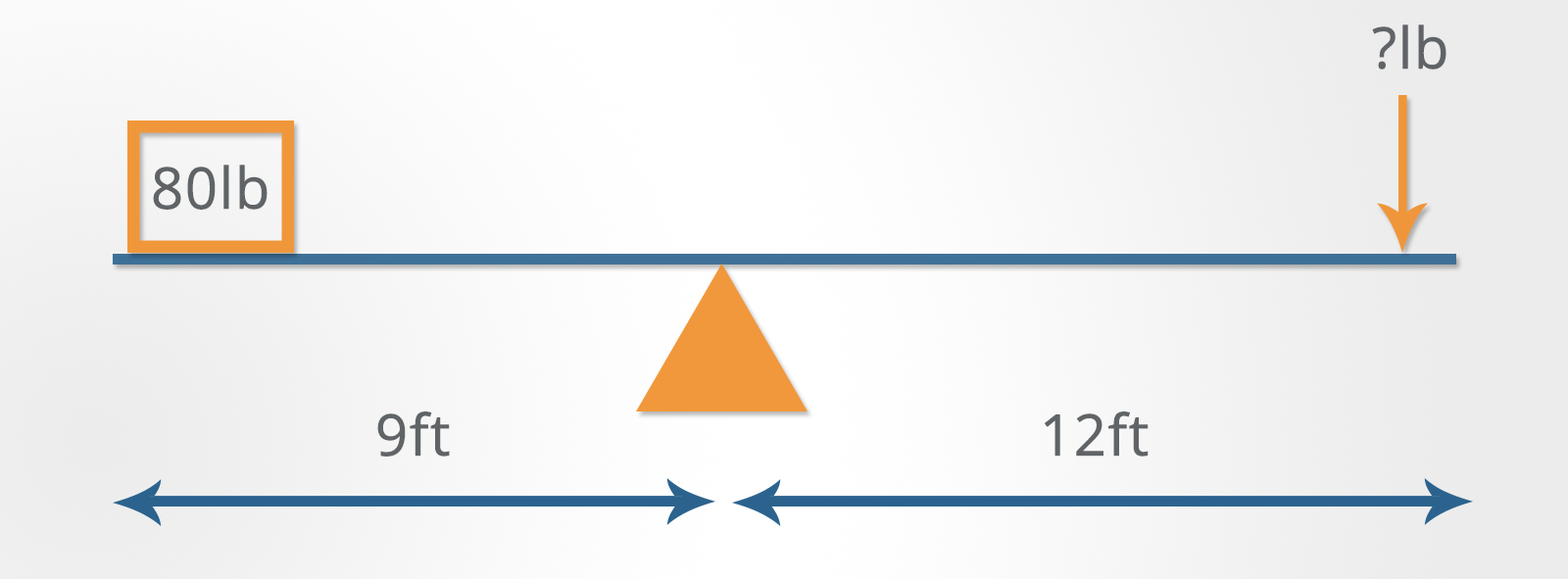Mechanical Reasoning Tests

1. How much force is required to lift the weight?

A) 40 lbs
B) 50 lbs
C) 60 lbs
D) 70 lbs

60 lbs are needed to lift the weight.

It can be calculated like this:

f = (w x d1) / d2
f = (80 x 9) / 12
f = (720) / 12
f = 60 lbs

2. How much force is required to lift the weights?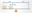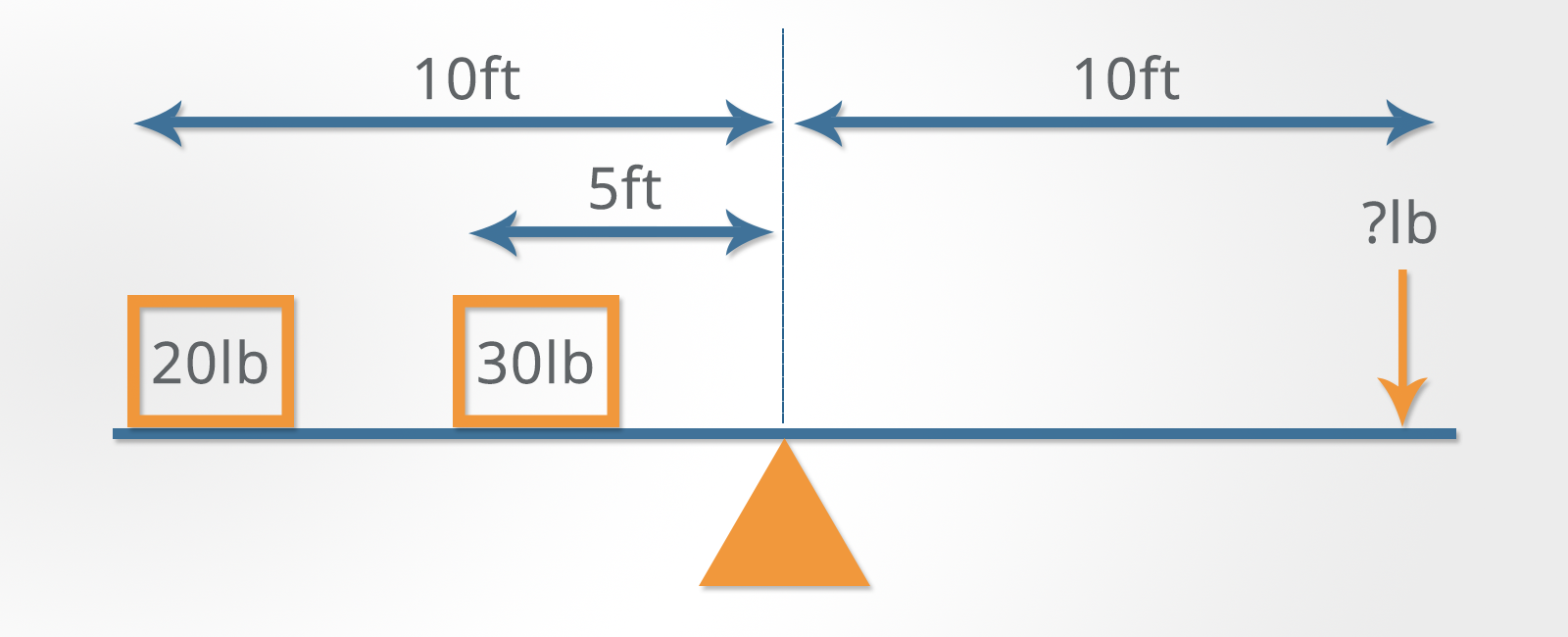Mechanical Reasoning Tests

A) 25 lbs
B) 35 lbs
C) 40 lbs
D) 45 lbs

35 lbs are needed to lift the weight.

It can be calculated like this:

f = (w1 x d1) + (w1a x d1a) / d2
f = (20 x 10) + (30 x 5) / 10
f = (200 + 150) / 10
f = 35 lbs

### Pulleys

The pulleys used in this type of question are made up of a grooved wheel and a block that holds it.

A rope runs in the groove around the wheel and one end will usually be attached to either:

• A weight
• A fixed object like the ceiling
• Another pulley

For these questions, you can ignore the effect of friction.

#### Single Pulley

Single pulley questions are relatively straightforward.

If the pulley is fixed, the force required is equal to the weight. If the pulley moves with the weight, the force is equal to half of the weight.

Another way of thinking about this is to divide the weight by the number of sections of rope supporting it to obtain the force needed to lift it.

Example question:

1. Which weight requires the least force to move?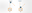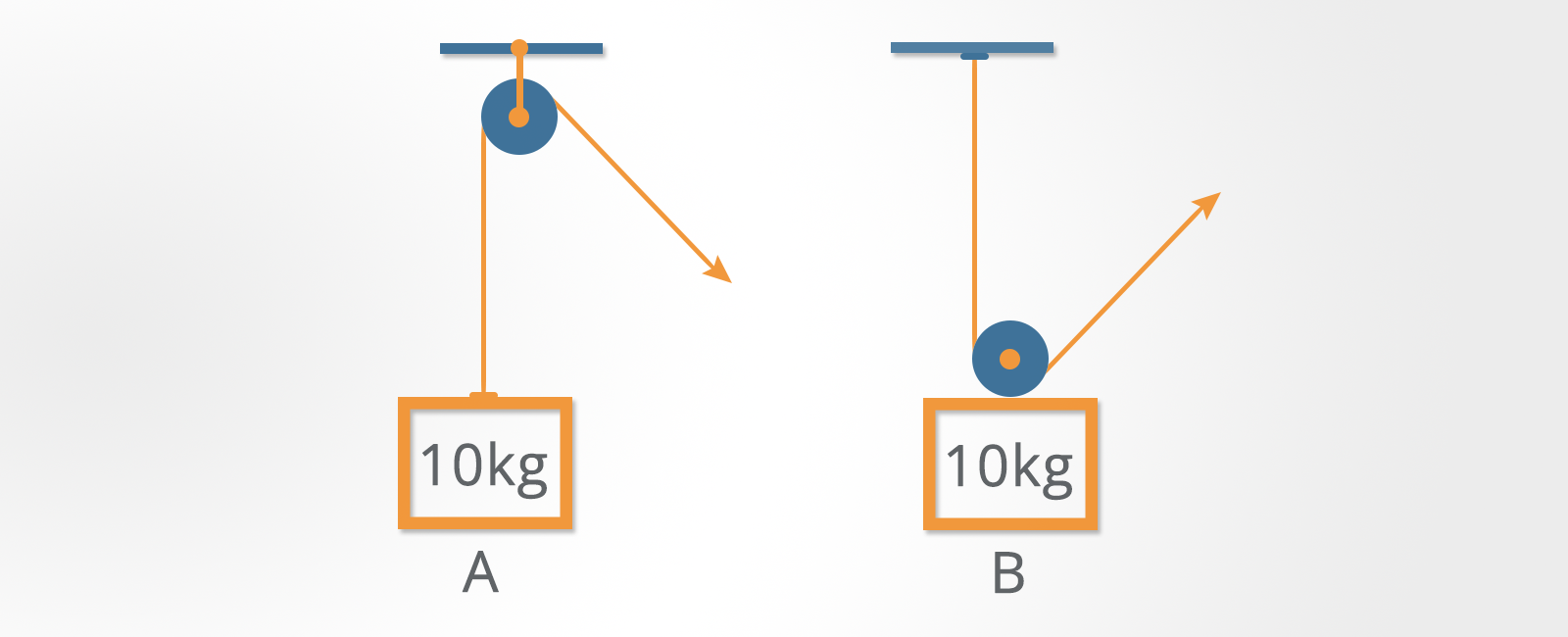Mechanical Reasoning Tests

Weight ‘B’ requires a force equal to 5 kg whereas ‘A’ requires a force equal to 10 kg.

In ‘A’ there is only one section of rope supporting the weight, so 10/1 = 10 kg required to lift the weight.

In ‘B’ there are two sections of rope supporting the weight, so 10/2 = 5 kg required to lift it.

#### Double Pulleys

There are two possible ways that two pulleys can be used. Either one pulley can be attached to the weight or neither of them can be.

Example question: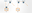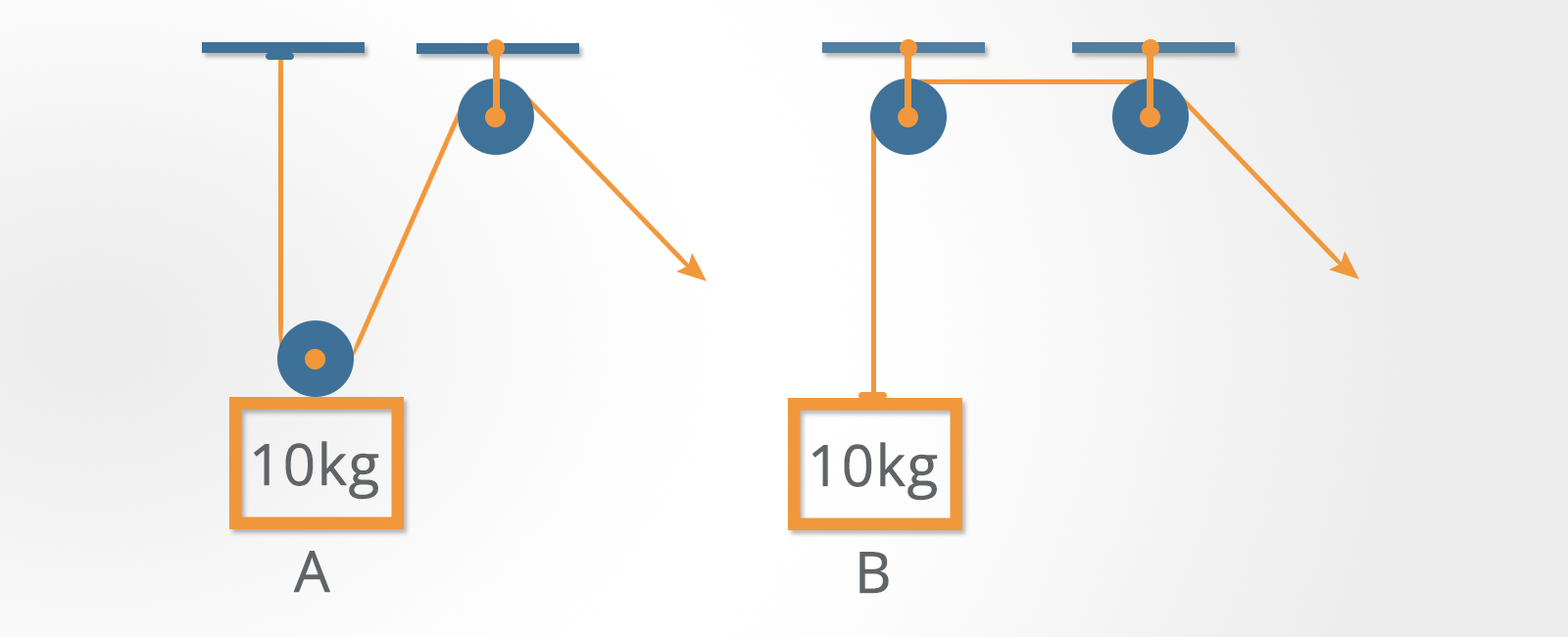Mechanical Reasoning Tests

1. Which weight requires the least force to move?

A) A
C) Both require the same force

Weight ‘A’ requires a force equal to 5 kg whereas weight ‘B’ requires a force equal to 10 kg.

Remember to divide the weight by the number of sections of rope supporting it to get the force needed to lift the weight.

#### Using More Than Two Pulleys

In all cases, just divide the weight by the number of sections of rope supporting it to get the force needed to lift the weight.

Example question:

1. How much force is required to move the weight?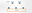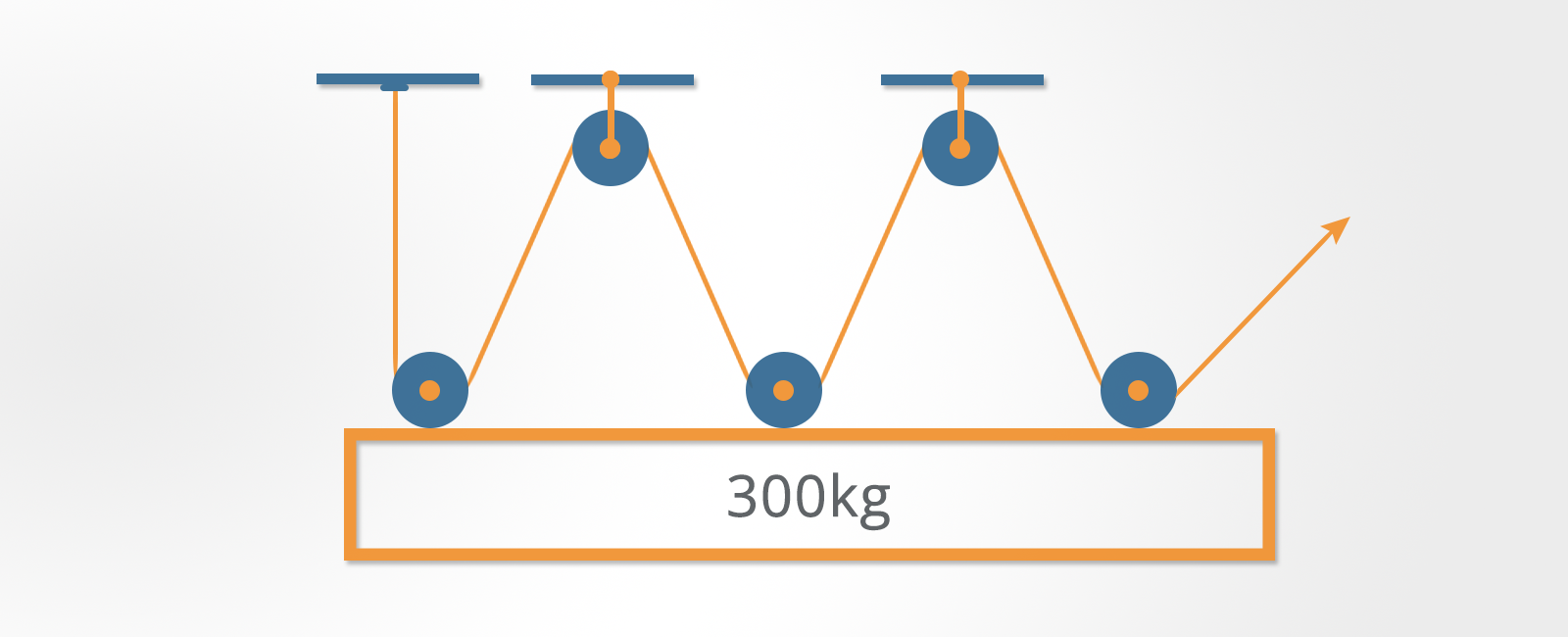Mechanical Reasoning Tests

A) 100 kg
B) 150 kg
C) 50 kg
D) 60 kg

The weight is 300 kg and there are six sections of rope supporting it. Divide 300 by 6 to get 50 kg.

### Gears

A gear is a toothed wheel or cylinder that meshes with another toothed component to transmit motion or to change speed or direction.

Gears are attached to a rotating shaft turned by an external force (which is not usually illustrated in these types of questions).

Two gears may be connected by touching each other directly or utilizing a chain or belt.

If gears are connected by a chain or belt then they move in the same direction.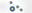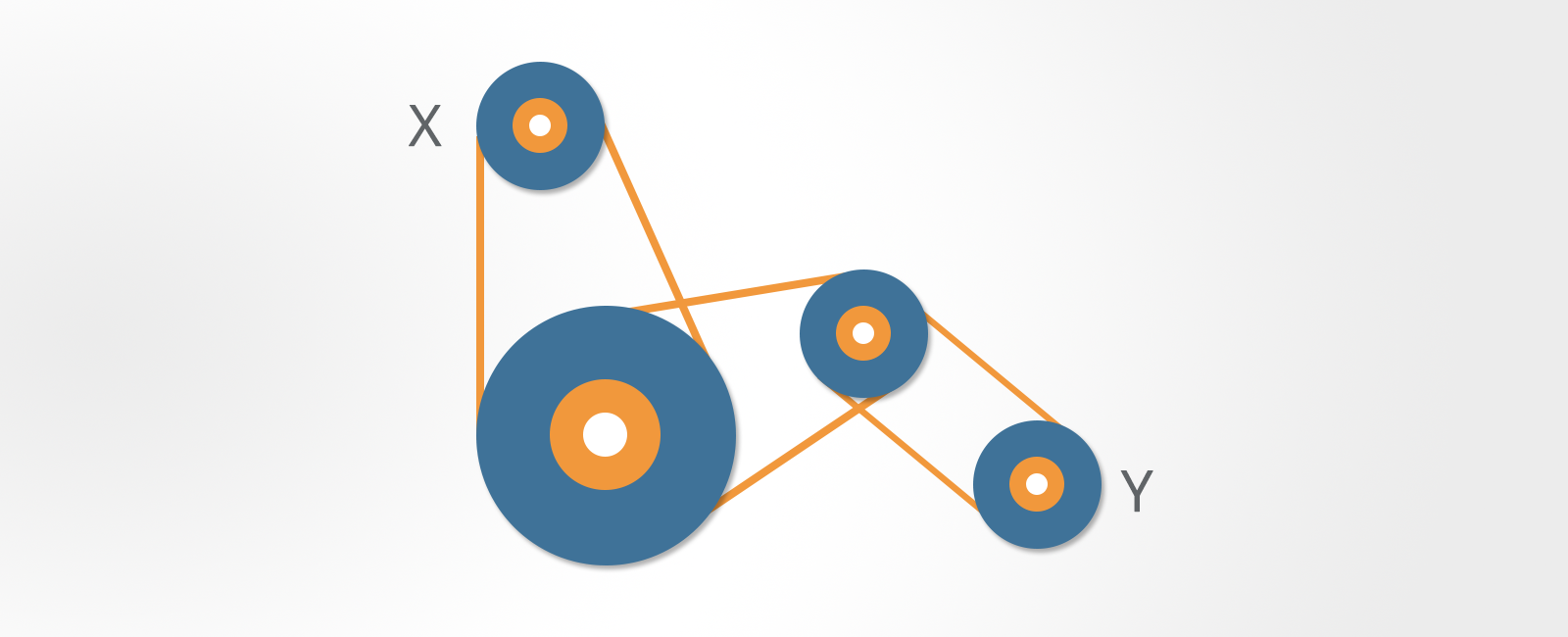Mechanical Reasoning Tests

If the gears are touching (meshed) then adjacent gears move in opposite directions.

In this example, the first and third gear will turn in the same direction.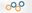Mechanical Reasoning Tests

When there is an odd number of meshed gears, the last gear will always turn in the same direction as the first one.

Meshed gears with an equal number of teeth will turn at the same speed. If they have an unequal number of teeth, the gear with the fewest teeth will turn faster.

To work out how fast one is turning with respect to the other you need to count the teeth.

Example questions:

1. If gear ‘X’ turns clockwise at a constant speed of 10 rpm, how does gear ‘Y’ turn?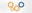Mechanical Reasoning Tests

A) anti c/w 10 rpm
B) c/w 10 rpm
C) c/w 5 rpm
D) anti c/w 5 rpm
E) c/w 20 rpm

2. If bar ‘Y’ moves left at a constant speed, how does bar ‘X’ move?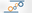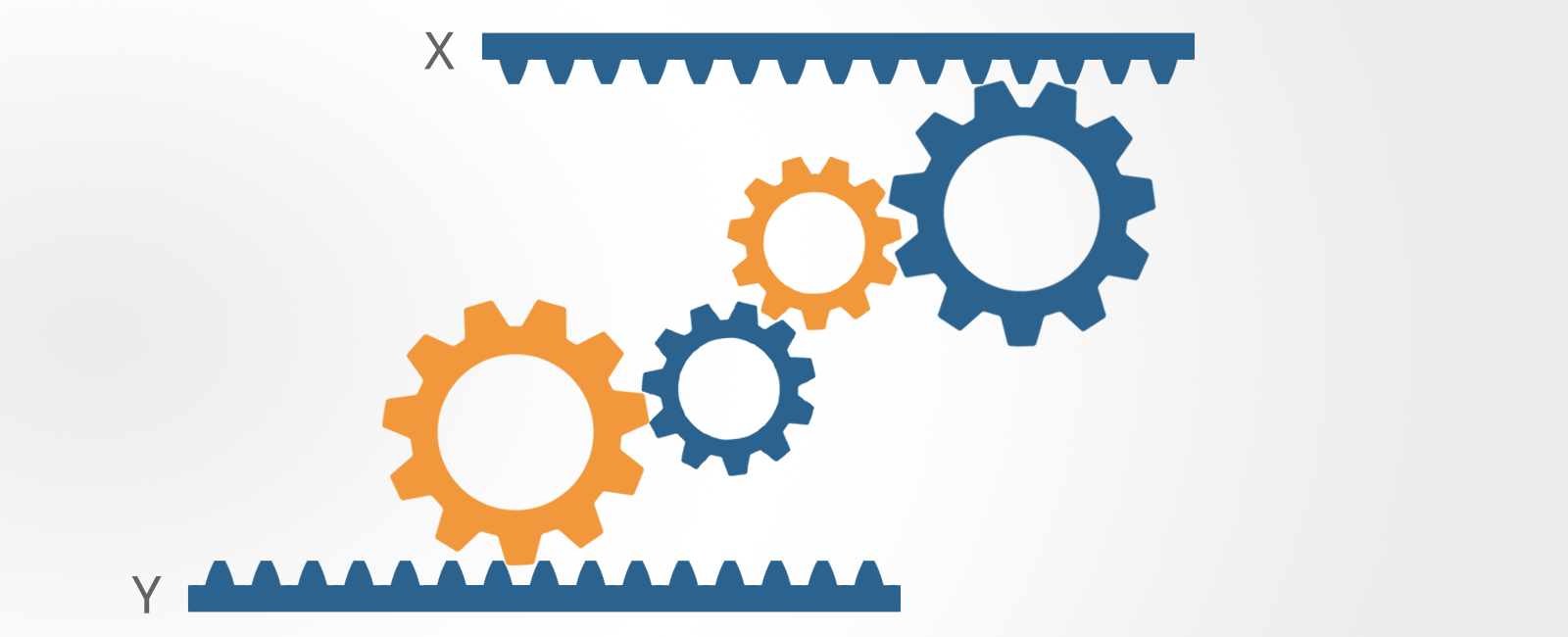Mechanical Reasoning Tests

A) Left, faster
B) Right, same
C) Left, slower
D) Left, same
E) Right, slower

3. If drive wheel ‘X’ rotates clockwise at a speed of 10 rpm, how does wheel ‘Y’ turn?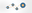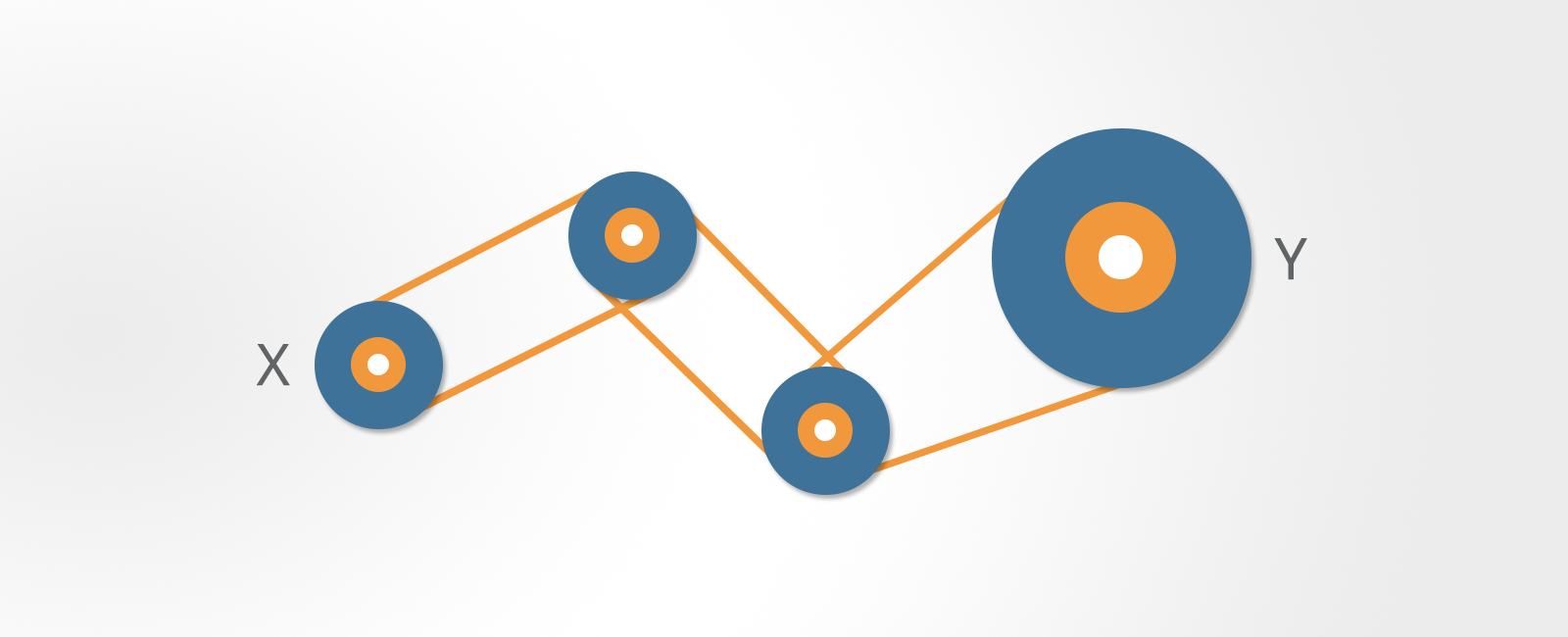Mechanical Reasoning Tests

A) anti c/w faster
B) c/w slower
C) c/w faster
D) anti c/w slower
E) anti c/w same

1. A
2. D
3. B

### Springs

A spring is a piece of wire or metal that can be extended or compressed by an external force but which then returns to its original length when that force is no longer applied.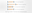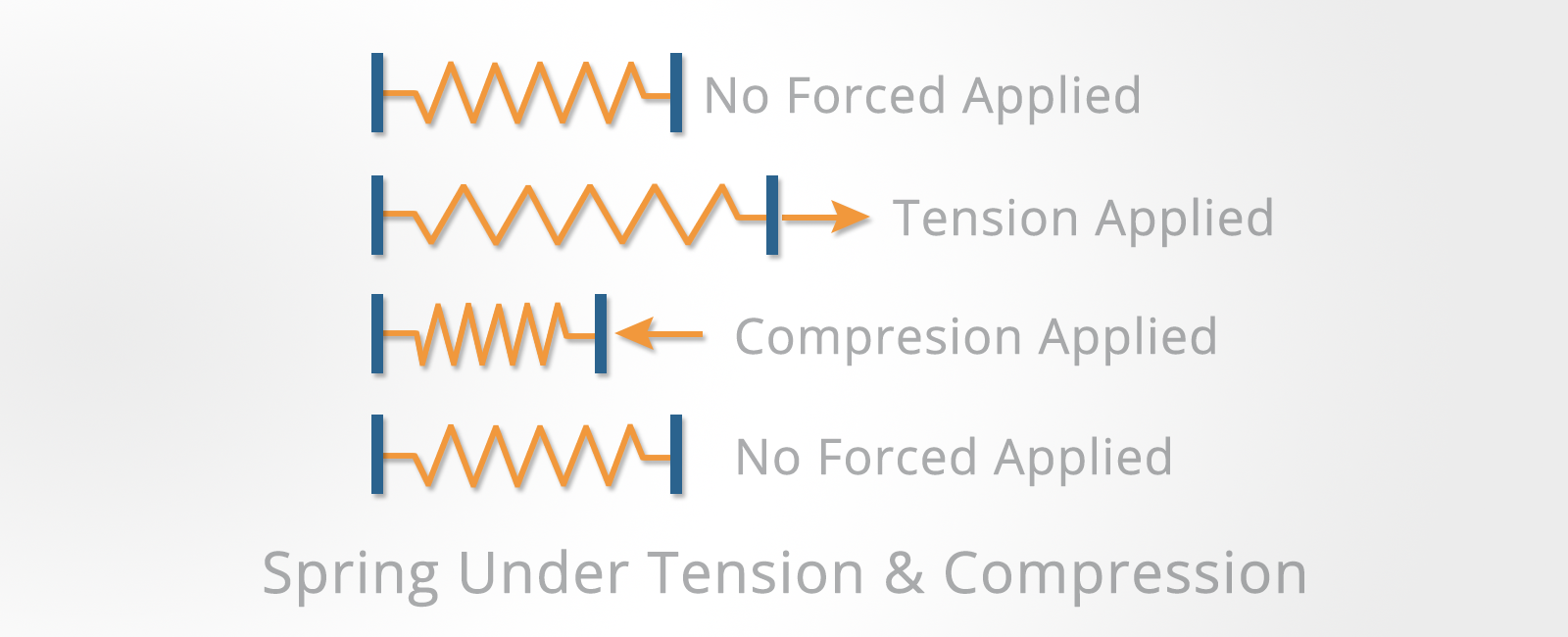Mechanical Reasoning Tests

There are many different types of spring, including spiral coil, leaf springs and torsion springs.

Springs are used in many applications including clocks, vehicle suspensions, etc.

In the type of questions that you will be asked in mechanical reasoning tests, you can assume that springs behave in a linear way.

That is, doubling the force applied will stretch or compress the spring twice as much.

#### Springs in Series and Parallel

If more than one spring is used then they can be arranged in series or in parallel.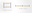Mechanical Reasoning Tests

When springs are arranged in series, each spring is subjected to the force applied.

When the springs are arranged in parallel, the force is divided equally between the springs.

Example question:

1. A force of 5 kg compresses the springs in series 10 cm. What will be the total distance that the springs in parallel are compressed?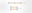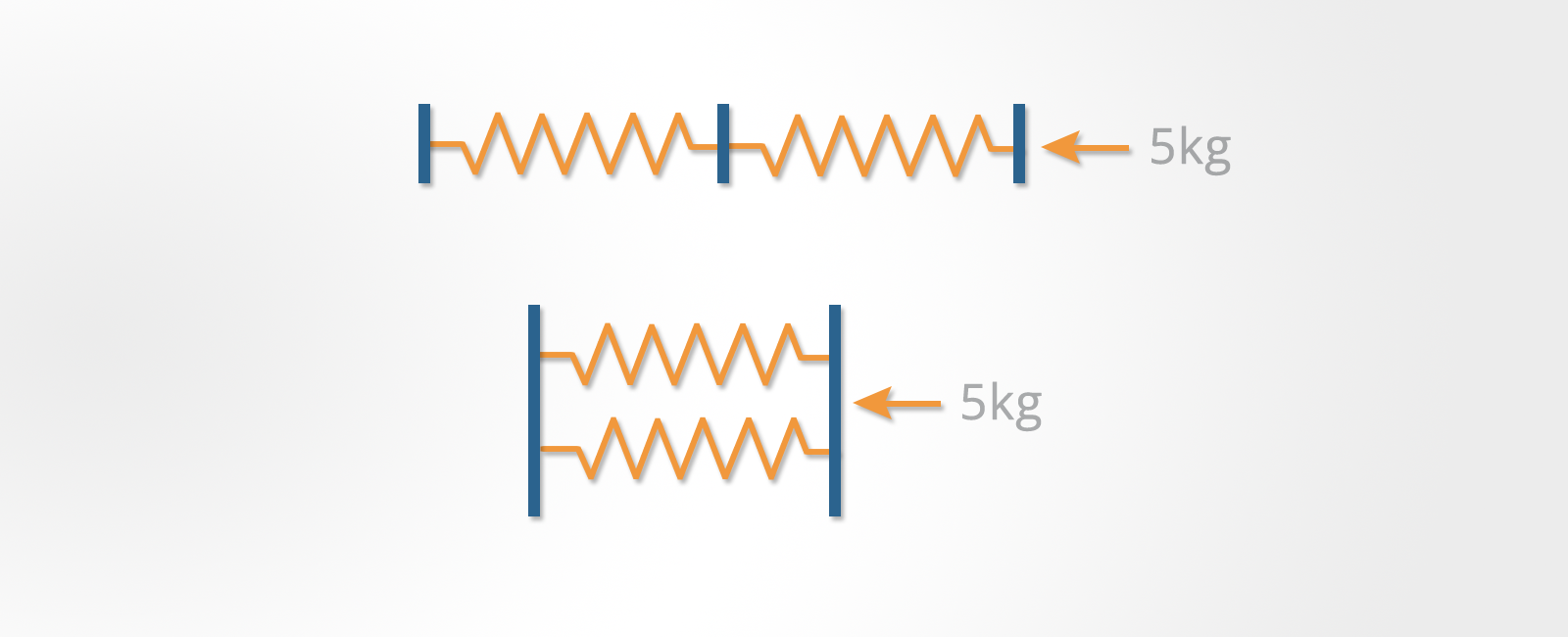Mechanical Reasoning Tests

A) 10 cms
B) 2.5 cms
C) 5 cms
D) 7.5 cms

The total force will be divided equally between the two springs in parallel.

Since the force is divided in half, the distance moved will also be halved. The springs in series were compressed 10 cms, so the springs in parallel will be compressed 5 cms.

### Simple Electrical Circuits

Questions on electricity usually take the form of simple circuit diagrams.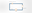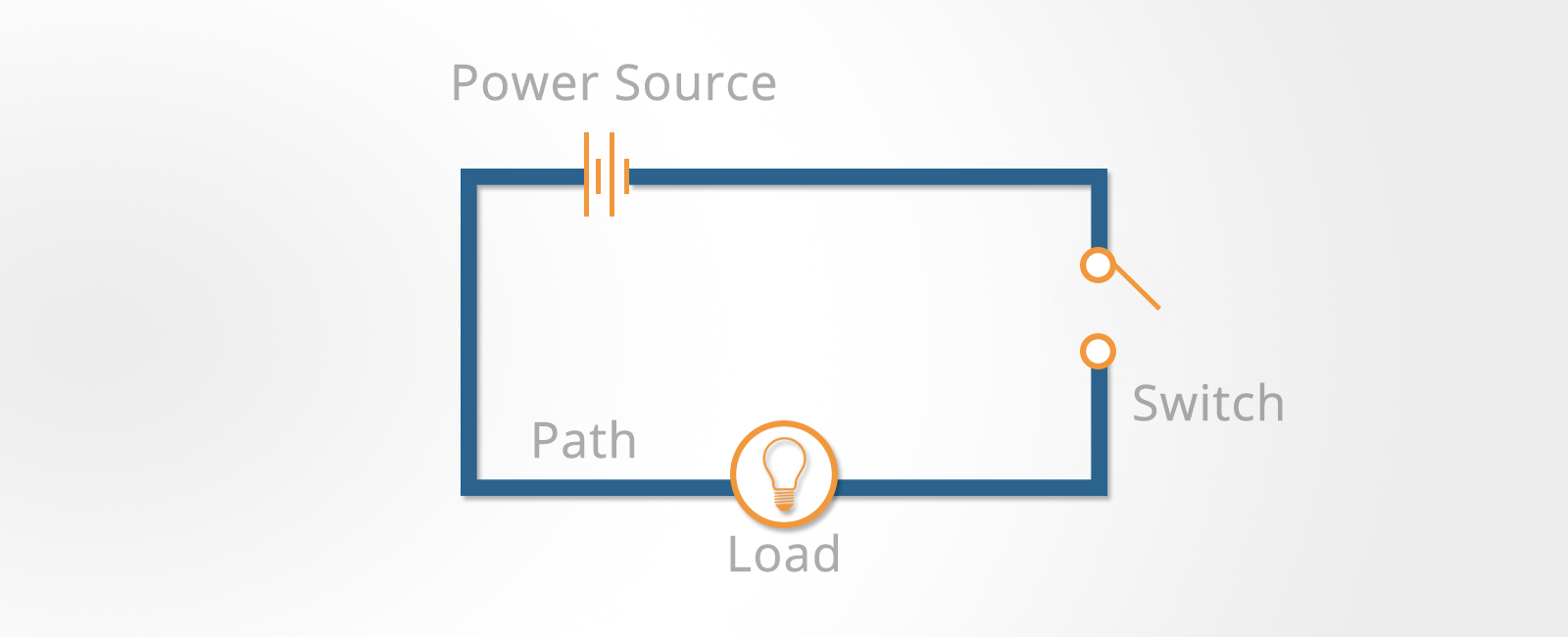Mechanical Reasoning Tests

These diagrams are usually restricted to showing:

• The power source
• Switches
• The path of the wiring

To answer these questions, you need a basic understanding of how electricity flows around a circuit.

Example questions:

1. How many switches need to be closed to light up one bulb?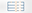Mechanical Reasoning Tests

A) 1
B) 2
C) 3
D) 4

2. How many bulbs will light up when the switch is closed?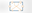Mechanical Reasoning Tests

A) 1
B) 2
C) 3
D) 4

3. If bulb 1 is removed, how many bulbs will light up when the switch is closed?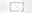Mechanical Reasoning Tests

A) 1
B) 2
C) 3
D) 4

1. B – Two switches need to be closed to complete a circuit
2. D – All four bulbs will light up
3. B – Only bulbs 2 and 4 will light up

### Tools

These questions are straightforward and if you have spent significant time fixing or making things, they won’t present any problems.

However, many people under 30 years of age don’t have much practical experience of fixing things or of watching someone else do so.

If you feel that this applies to you, you will need to make some effort to improve your knowledge of everyday tools.

You can do this by getting hold of a catalog for a tool hire company and simply reading through it – boring but effective.

Example questions: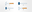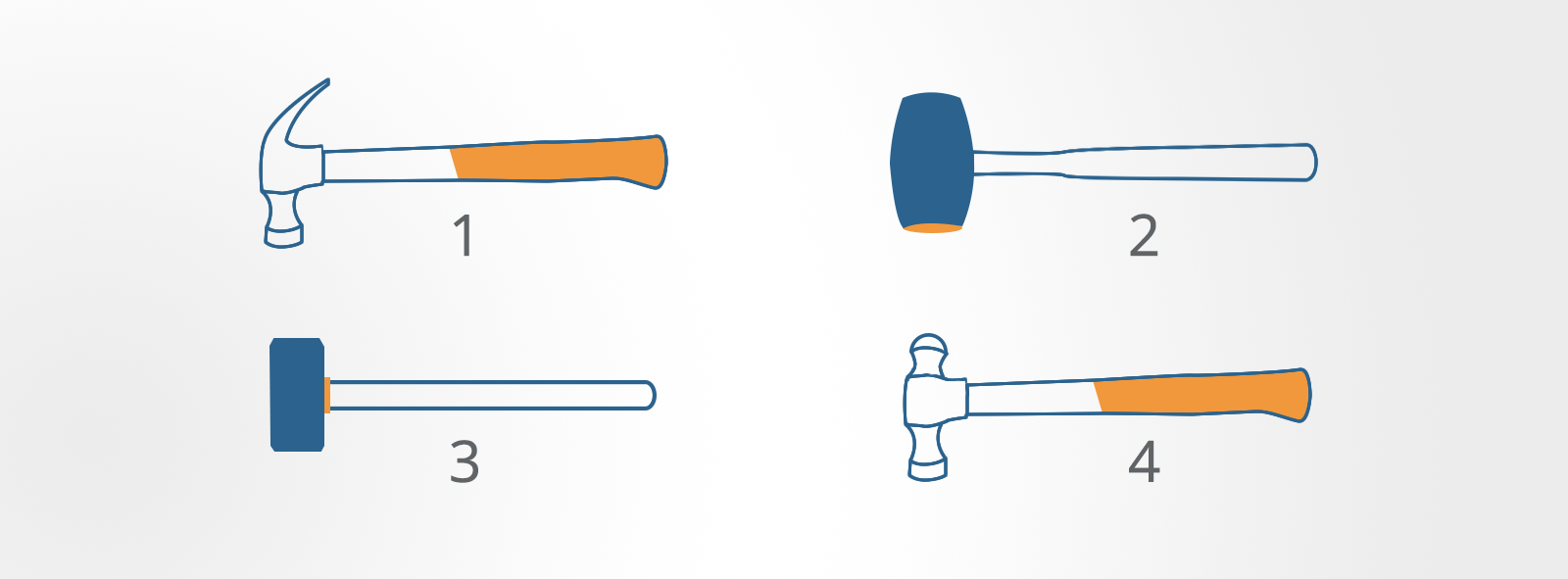Mechanical Reasoning Tests

1. Which hammer is the most suitable tool for general carpentry?

A) None
B) 1
C) 2
D) 3
E) 4

2. Which hammer is the most suitable tool for general metalwork?

A) None
B) 1
C) 2
D) 3
E) 4

3. Which is the most suitable tool for breaking up concrete?

A) None
B) 1
C) 2
D) 3
E) 4

4. Which is the most suitable tool for assembling a friction fit wooden frame?

A) None
B) 1
C) 2
D) 3
E) 4

1. B – Claw hammer
2. E – Ball-peen hammer
3. D – Sledgehammer
4. C – Rubber mallet

### Fault Diagnosis

Fault diagnosis tests are used to select technical personnel who need to be able to find and repair faults in electronic and mechanical systems.

As modern equipment of all types become increasingly complex and more dependent on electronic control systems, the ability to approach problems logically in order to find the cause of the fault is increasingly important.

This skill is critical when working with complicated systems like those found in aircraft, ships and communications equipment.

This type of test usually forms part of a test battery in which verbal aptitude and numerical aptitude are also tested.

There are two components to fault diagnosis questions:

1. A grid that describes the function of a series of switches
2. A flowchart showing: input – switches – output

Each of the switches acts to alter the input before the next switch in the series.

Example question: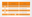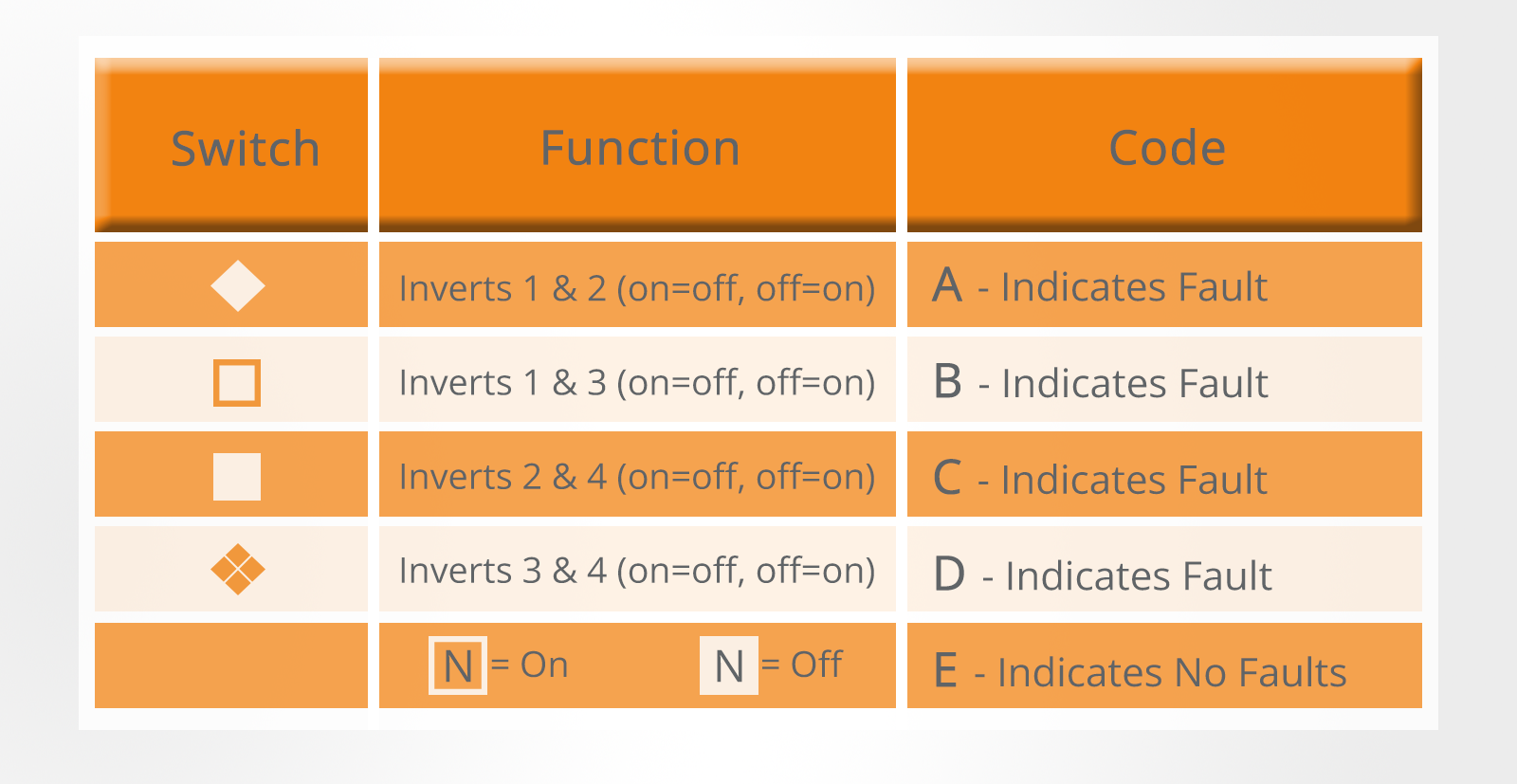Mechanical Reasoning Tests

This grid shows four switches and their effect on four numbered inputs which may be either ‘on’ or ‘off’.

The first switch (diamond symbol) inverts inputs one and two.

That is, if the input is on, it is turned off and if it is off, it is turned on.

The other switches act in a similar way as detailed in the grid.

If a switch is not working then it does not affect the input which passes through unchanged.

You need to diagnose where the fault is in the flowchart below: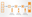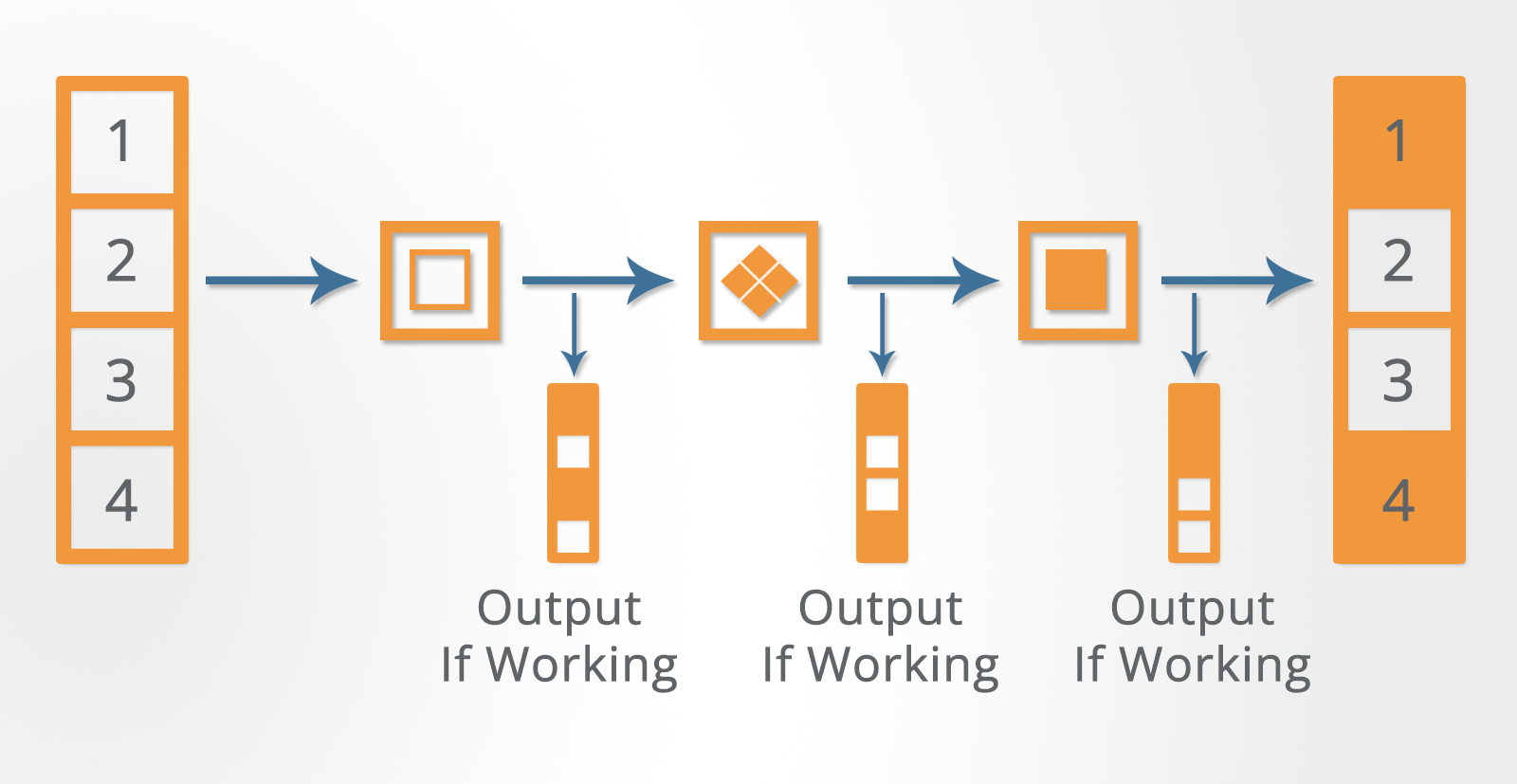Mechanical Reasoning Tests

In this flowchart, all of the inputs (numbered 1, 2, 3, 4) are on.

When these inputs pass through the first switch, 1 & 3 are inverted (i.e. switched off).

These modified inputs (1=off, 2=on, 3=off, 4=on) then pass through the second switch.

The second switch inverts inputs 3 & 4, which gives (1=off, 2=on, 3=on, 4=off).

These modified inputs (1=off, 2=on, 3=on, 4=off) then pass through the third switch.

The third switch should invert inputs 2 & 4, giving (1=off, 2=off, 3=on, 4=on).

However, the third switch is not working so the output from switch two is not changed.

Answer: C – because switch type ‘C’ is at fault.

## How to Pass Mechanical Reasoning Questions – Top Tips

Mechanical reasoning tests will measure your applied knowledge so revision and practice are essential.

It doesn’t matter if the questions you practice on aren’t specific to the industry you are applying for. It is the substance of the question that is important – the scenario is incidental.

Don’t just focus on practice tests, either. Mechanical aptitude is, in large part, gained by practical application. It is a good idea to look at and experiment safely with real tools and learn how things go together and are taken apart. Read user manuals and try things out.

Need further practice? If so, we recommend the mechanical test packages by JobTestPrep.

## Conclusion

Mechanical reasoning tests measure what you have learned about mechanical concepts, tools, physics, arithmetic and fault diagnosis.

To get a good score, practice is essential. Work through the practice questions provided by Psychometric Success and you will be well prepared for your test.